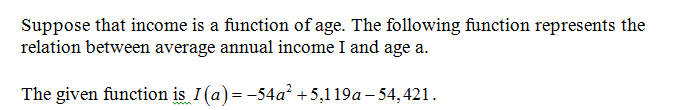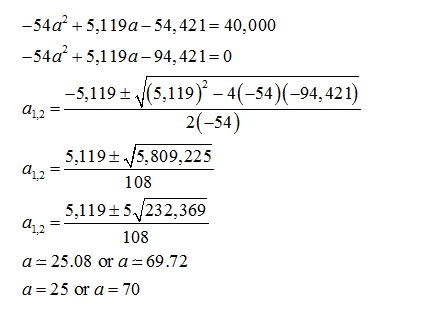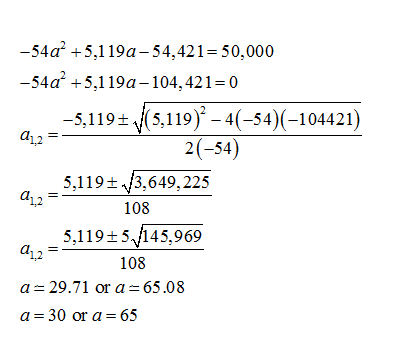# Suppose that income is a function of age. The following function represents the relation between average annual income I and age a. I(a)=-54a^2 +5,119a -54,421 ​(a) For what age does average income I equal \$40,000?​(b) For what age does average income I equal \$50,000?

Question
30 views
Suppose that income is a function of age. The following function represents the relation between average annual income I and age a.

I(a)=-54a^2 +5,119a -54,421

​(a) For what age does average income I equal \$40,000?
​(b) For what age does average income I equal \$50,000?
check_circle

(a) Given:Find for what age does average income I equal \$40,000.(b) Find for what age does average income I equal \$50,000....

### Want to see the full answer?

See Solution

#### Want to see this answer and more?

Solutions are written by subject experts who are available 24/7. Questions are typically answered within 1 hour.*

See Solution
*Response times may vary by subject and question.
Tagged in